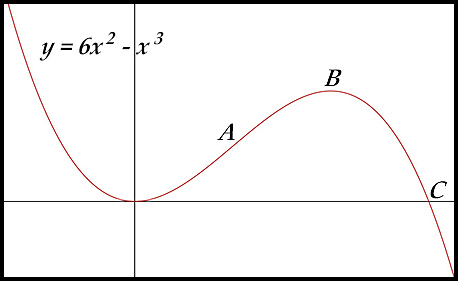Dr. J's Maths.com
Where the techniques of Maths
are explained in simple terms.

Calculus - Differentiation - Applications of Calculus.
Given an equation - Test Yourself 2.

 The questions of this page focus on the following issues: 1. Finding stationary points. 2. Where is a curve increasing/decreasing. 3. The concavity of the curve. 4. The points of inflexion. 5. The number of solutions for an equation.

 Stationary points and sketching. 1. A function is defined as f(x) = x3 - 3x2. (i) Find the coordinates of the stationary points and determine their nature. (ii) Find the coordinates of the point of inflexion. (iii) Sketch the graph of y = f(x) indicating clearly the stationary points, the point of inflection and the x - intercepts. (iv) Find the minimum value of the function in the interval [-2, 3]. Answer. (i) Maximum at (0,0);minimum at (2, -4).(ii) POI at (1, -2). (iv) Minimum is -20. 2. For the cubic function y = x3 - x2 - x + 6 (i) find the coordinates of the stationary points and determine their nature. (ii) Find any point(s) of inflexion. 3. The function f(x) = 2x3 + 3x2 - 12x + 7 is defined with the domain [-3. 3]. (i) Find the stationary points and determine their nature. (ii) Find the point of inflexion. (iii) Sketch the graph of the function in the given domain indicating all main points (but not the x-intercepts). (iv) What is the maximum value in the given domain? (v) Find the values for x where the first derivative is less than 0 and the second derivative is greater than 0. Answer. (i) Maximum at (-2,27);minimum at (-1, 0). (ii) POI at (-0.5, 13.5). (iv) Max value in domain is 52.(v) -0.5 < x < 1. 4. The function f(x) = x3 - 6x2 + 9x -4 is defined in the domain [0, 4]. (i) Find the coordinates of any stationary point and determine their nature. (ii) Draw a neat sketch and indicate the approximate position of any points of inflection. . 5. Given that f(x) = (x - 2)2(3 - x): (i) Expand the terms to show that f(x) can be expressed as: f(x) = -x3 +7x2 - 16x + 12 (ii) Find the stationary points for the function and determine their nature. (iii) Draw a sketch of y = f(x) showing the stationary points and the intercepts. Answer. (ii) Maximum at (8/3,4/27);minimum at (2, 0). (iIi) Intercepts at (2, 0) andat (0, 12). Absolute maximum & minimum values Curve increasing/decreasing. 6. (i) For the curve y = 3x - x3, find the stationary points and determine their nature. (ii) For what values of x is the curve increasing? Answer. (i) Maximum at (1,2); minimum at (-1, -2). (ii) Increasing for -1 < x < 1. Concavity 7. A function is defined by f(x) = x3 - 3x2 - 9x - 22. (i) Find the coordinates of the turning points of the function f(x) and determine their nature. (ii) Find the coordinates of the points of inflection. (iii) Sketch the graph of y = f(x) showing the main features. (iv) For what values of x is the graph of y = f(x) concave up? Answer. (i) TPs at (-1, 27) max and(3, -5) min; (ii) POI at (1, 11).(iv) x > 1. 8. For what values of x is the curve f(x) = 2x3 + x2 concave down? Answer. Concave down for x < 1/6. Points of inflexion. 9. The diagram below shows a sketch of the curve y = 6x2 - x3.The curve cuts the x-axis at C. It also has point of inflexion at A and a local maximum at B. (i) Find the coordinates of C. (ii) Find the coordinates of B. (iii) Find the coordinates of A. 10. Number of solutions. 11. 12.